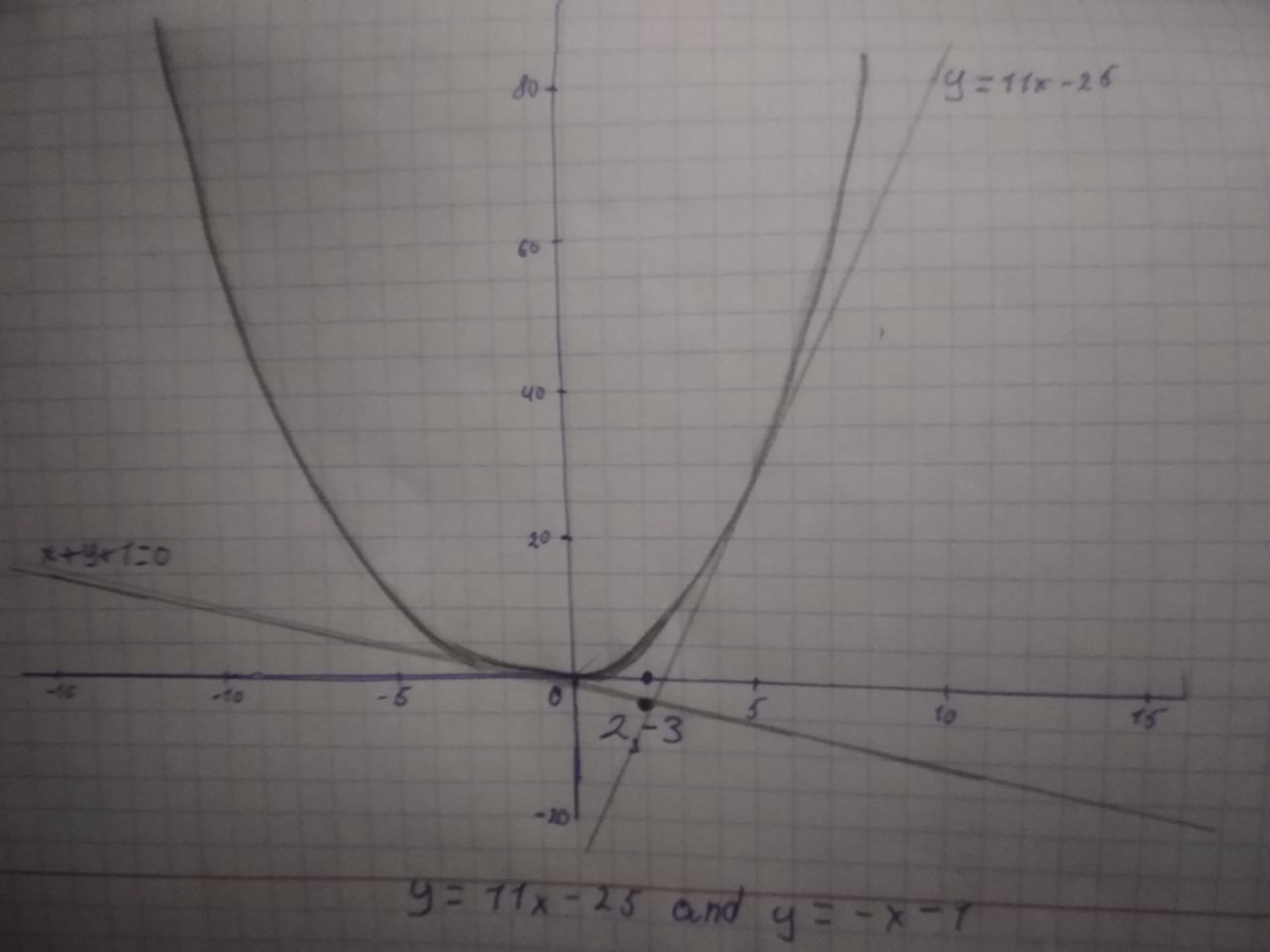#### Didn’t find what you are looking for?

Question# Find equations of both lines through the point (2, −3) that are tangent to the parabola y = x^2 + x.

Linear equations and graphs
ANSWEREDFind equations of both lines through the point (2, −3) that are tangent to the parabola $$y = x^2 + x$$.2021-05-24

Note that all points on the parabola $$y = x^2 + x$$ are of the form $$(x, x^2 + x)$$
Assume that the tangent at $$(a, a^2 + a)$$ passes through (2, -3)
We know that the slope of tangent at any point is the derivative at that point.
Differentiate $$y = x^2 + x, To\ get \frac{dy}{dx} = 2x + 1$$
Therefore, the slope of tangent at $$(a, a^2 + a) is 2a + 1 \rightarrow (1)$$
Since the tangent passes through the points $$(a, a^2 + a)$$ and (2. -3). We can write the slope of the tangent as
$$\frac{(a^2 + a)-(-3)}{a - 2} = \frac{a^2 + a + 3}{a - 2} \rightarrow (2)$$
Using (1) and (2), we can write $$\frac{a^2 + a + 3}{a - 2} = 2a + 1$$
$$a^2 + a + 3 = (2a + 1)(a - 2)$$
$$a^2 + a + 3 = 2a^2 + a - 4a - 2$$
$$a^2 - 4a - 5 = 0$$
$$(a + 1)(a - 5) = 0 \Rightarrow a = -1\ and\ a = 5$$
Therefore, there are two tangents that pass through (2, -3)
Finding the equation of the tangent corresponding to $$a = -1$$
Note that the slope of the tangent is $$2a + 1 = 2(-1) + 1 = -1$$
Since the tangent. passes through (2, -3).
Therefore, the equation of the tangent is
$$\frac{y - (-3)}{x - 2} = -1$$
$$y + 3 = -(x - 2)$$
$$x + y + 1 = 0$$
Finding the equation of the tangent corresponding to $$a = 5$$
Note that the slope of the tangent is $$2a + 1 = 2(5) + 1 = 11$$
Since the tangent. passes through (2, -3).
Therefore, the equation of the tangent is
$$\frac{y - (-3)}{x - 2} = 11$$
$$y + 3 = 11(x - 2)$$ $$y = 11x - 25$$